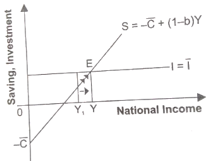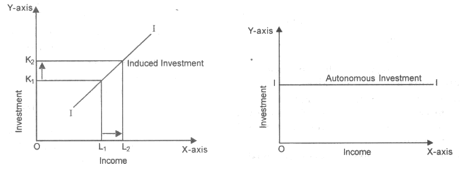# Determination of Income and Employment Class 12 Economics Extra Questions## myCBSEguide App

Complete Guide for CBSE Students

NCERT Solutions, NCERT Exemplars, Revison Notes, Free Videos, CBSE Papers, MCQ Tests & more.

Determination of Income and Employment Class 12 Economics Extra Questions. myCBSEguide has just released Chapter Wise Question Answers for class 12 Economics. There chapter wise Practice Questions with complete solutions are available for download in myCBSEguide website and mobile app. These test papers with solution are prepared by our team of expert teachers who are teaching grade in CBSE schools for years. There are around 4-5 set of solved Economics Test Papers from each and every chapter. The students will not miss any concept in these Chapter wise question that are specially designed to tackle Board Exam. We have taken care of every single concept given in CBSE Class 12 Economics syllabus and questions are framed as per the latest marking scheme and blue print issued by CBSE for class 12.

CBSE Class 12 Economics Extra Questions

## Practice Questions for Class 12 Economics

Determination of Income and Employment

1. In S = – a + (1 – b) Y, ‘a’ denotes (1)

1. Automatic consumption expenditure
2. Annual consumption expenditure
3. Autonomous savings.
4. Consumption expenditure
2. MPC = 1 – MPS. It is (1)

1. None of these
2. True.
3. Depends on their values
4. False.
3. If MPC is less than one, it follows that (1)
1. (1-b) i.e. MPS is zero
2. (1-b) i.e. MPS is positive
3. (1-b) i.e. MPS is negative
4. (1-b) i.e. MPS is infinity
4. The formula of investment multiplier in terms of MPS is (1)

1. {tex}\frac{{\Delta C}}{{\Delta D}}{/tex}
2. {tex}1 \over MPS{/tex}
3. {tex}\frac{C}{{\Delta I}}{/tex}
4. {tex}\frac{C}{Y}{/tex}
5. Why should price rise only after full employment? (1)

6. If planned saving are greater than planned investments, what will be its effect on inventories? (1)

7. What is full employment? (1)
8. Categorise the following as induced investment and autonomous investment. (1)

1. Government has set up public health centres in rural areas.
2. Government has decided to invest Rs. 1,000 crore to save Sunder ban Forests.
3. Airtel has invested ? 1,000 crore to improve its services.
9. Calculate the equilibrium level of income in the economy. (3)
C = 500 + (0.9)Y;
Investment expenditure = 3,000

10. From the following data calculate investment expenditure: (3)
Marginal Propensity to Save = 0.2
Equilibrium level of income = Rs 22,500
Autonomous consumption = Rs 500.

11. What is deficient demand in an economy? What is its impact on output, employment and prices? (4)

12. Calculate Autonomous Consumption expenditure from the following data about an economy which is in equilibrium: (4)
National Income = Rs 1,200
Marginal Propensity to Save = 0.20
Investment expenditure = Rs 100

13. If planned saving is less than planned investment, what changes will bring economy in equilibrium? (4)

14. Differentiate induced and autonomous investment. Explain and show them with the help of a diagram also? (6)

15. Calculate investment expenditure from the following data about an economy which is in equilibrium. (6)
National Income = Rs 1,000
Marginal Propensity to Save = 0.20
Autonomous consumption expenditure = Rs 100

Determination of Income and Employment

1. Autonomous savings.
Explanation: ‘a’ is constant which implies that this saving takes place even when income is zero and is independent of the level of income.So, ‘a’ denotes autonomous savings.
1. True.
Explanation:
MPS + MPC = 1
MPC = 1 – MPS
1. (1-b) i.e. MPS is positive
Explanation: b stands for MPC. So, when b is less than 1, evidently 1-b is positive or 1-b>0
1. {tex}1 \over MPS{/tex}Explanation: {tex}1 \over MPS{/tex}
1. Price level do not rise before full employment because AS is assumed to be perfectly elastic. Whatever be the level of aggregate demand, AS converges with AD so that AD and AS tends to be equal without causing any change in price level. But when AD increases beyond its full employment level, then the mounting pressure of demand on the existing output casuses a rise in prices.
2. The inventories will rise.
3. It is a situation in which all those people who are able and willing to work at the prevailing wage rate get work.
4. i.&ii .Autonomous Investment: Establishment of public health centres and governments investment on saving Sunder ban forests. These investments are not done with profit motive.iii.Induced Investment : Airtel investment is driven by profit motive.
5. Given C = 500 + (0.9)Y,
Investment expenditure, (I) = Rs.3,000;
At equilibrium level, Y = C + I
{tex} \Rightarrow {/tex} Y = 500 + (0.9) Y+ 3,000
{tex} \Rightarrow {/tex} Y – (0.9)Y = 3,500 => (0.1)Y = 3,500{tex}Y = \frac { 3,500 } { 0 .1 } = 735,000{/tex}. Therefore equilibrium level of Income = Rs. 735,000.
6. Given, Marginal Propensity to Save (MPS ) = 0.2, Income (Y) = 22,500, {tex}\overline { C } = 500{/tex},
We know that, MPC = 1 – MPS = 1 – 0.2 = 0.8.
At equilibrium level,
Y = C + I
Therefore 22,500 = 500 + (0.8) 22,500 + I
Or I = 22,500 – 500 – 18,000 = Rs.4,000. Hence we have Investment Expenditure equal to Rs. 4000.
7. Deficient demand refers to the situation when AD is short of AS corresponding to the full employment level in the economy.
1. Effect on output : Low level of investment and employment implies low level of output.
2. Effect on employment : Because of deficiency of demand, investment level is reduced. Accordingly, level of employment tends to fall.
3. Effect on prices : Fall in prices is the immediate consequence of deficient demand.
8. Calculation of Autonomous Consumption {tex}( \overline { C } ){/tex}:Given,
1. National Income (Y) = Rs 1,200
2. Investment Expenditure (I) = Rs 100,
3. Marginal Propensity to Save (MPS) = 0.20

Calculation of Marginal Propensity to Consume (MPC or b)
= 1 – Marginal Propensity to Save(MPS)
=1-0.20 = 0.80
{tex}\therefore{/tex} MPC = 0.80
Also, Y = C + I, or Y {tex}= \overline { C } + b Y + I{/tex} ….. (i)
{tex}\because \quad C = \overline { C } + b Y{/tex}
On substituting the given variables in equation (i), we get
{tex}1,200 = \overline { \mathrm { C } } + 080 \times 1,200 + 100{/tex}
{tex}1,200 = \overline { C } + 960 + 100{/tex}
{tex}= \overline { \mathrm { C } } + 1,060{/tex}
{tex}\therefore \overline { C }{/tex}= 1,200 – 1,060 = Rs 140
{tex}\therefore{/tex} Autonomous consumption expenditure {tex}( \overline { C } ) = Rs \ 140{/tex}

C + I = C + S
I = C + S -C = S

1. when planned saving is less than planned investment, then national income will decrease as shown in the below diagram.2. When, investment > saving [at Y1], then production will have to be increased to meet the excess demand. Consequently, national income will increase leading to rise in saving until saving becomes equal to investment. It is here that equilibrium level of income is established because what the savers intend to save becomes equal to what the investors intend to invest.
1. Induced Investment:
1. Induced investment is positively related to the level of income in an economy. At higher level of income, consumption expenditure tends to increase. Increased consumption expenditure or level of demand raises expected profitability of the producer who accordingly are induced to make greater investment.
2. Induced investment curve has a positive slope showing direct relationship between income and investment.
2. Autonomous Investment :
An investment which is not influenced by expected profitability or level of income is called autonomous investment. In fact, it is an investment expenditure incurred by the government with a view to promoting the level of aggregate demand in the economy when AD falls short of AS, resulting in fall in prices and rise in unemployment. The govt. intends in push up the level of AD by way of its autonomous investment. Autonomous investment is independent of the level of income. It is also profit and income inelastic.10. Given, National Income (Y) = 1,000,
Marginal Propensity to Save (MPS) = 0.20,
Autonomous Consumption {tex}(\overline C ){/tex} = 100
MPC or b = 1 – MPS = 1 – 0.20 = 0.80
Y = C + I
C = {tex}\overline C {/tex} + b(Y)
∴ Y = {tex}\overline C {/tex} + b(Y) + I
∴ On substituting the given variables above, we get
{tex}1,000 = 100 + 0.80 \times 1,000 + I{/tex}
I = 1000 – 900 = 100
i.e. Investment = Rs 100

## Chapter Wise Practice Test for Class 12 Economics## Test Generator

Create Papers with your Name & Logo